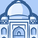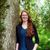# QlikView App Dev

Discussion Board for collaboration related to QlikView App Development.

Announcements
Join this live chat April 6, 10AM EST - QlikView to Qlik Sense REGISTER
cancel
Showing results for
Search instead for
Did you mean:Creator II

## Using different expression based upon a box selection

Dear Qlikview user

I am not sure how to go about this, but I want to create an expression for when a user has a selection on FiscalYear, the text box will display one expression, but as soon as Month is selected also, a different expression displays

I do not know whether I would need 2 text boxes on a show/hide scenario, or whether Qlikview has an already built in function which allows you to change expressions based on the users selections

I.e.

If(Fiscal Year is selected then,

Num(sum({\$<Week_Number,Month,QuarterName,FiscalYear,FactDateNum=P(FactDateNum_prevYear)>} If(RTT_WEEKS_WAIT <'18', RTT_COUNTER))/

sum({\$<Week_Number,Month,QuarterName,FiscalYear,FactDateNum=P(FactDateNum_prevYear)>}RTT_COUNTER), '0.00%'),

If(Fiscal Year and Month is selected Then,

Num(sum({\$<Week_Number,Month,QuarterName,FiscalYear,FactDateNum=P(FactDateNum_prevMonth)>} If(RTT_WEEKS_WAIT <'18', RTT_COUNTER))/

sum({\$<Week_Number,Month,QuarterName,FiscalYear,FactDateNum=P(FactDateNum_prevMonth)>}RTT_COUNTER), '0.00%'),

Am I looking to use something like:

GetCurrentField

GetCurrentSelections

Any help would be greatly appreciated

Kind Regards

Helen

1 Solution

Accepted SolutionsMVP

If(GetSelectedCount(FiscalYear) > 0,

Num(sum({\$<Week_Number,Month,QuarterName,FiscalYear,FactDateNum=P(FactDateNum_prevYear)>} If(RTT_WEEKS_WAIT <'18', RTT_COUNTER))/

sum({\$<Week_Number,Month,QuarterName,FiscalYear,FactDateNum=P(FactDateNum_prevYear)>}RTT_COUNTER), '0.00%'),

If(GetSelectedCount(FiscalYear) > 0 and GetSelectedCount(Month) > 0,

Num(sum({\$<Week_Number,Month,QuarterName,FiscalYear,FactDateNum=P(FactDateNum_prevMonth)>} If(RTT_WEEKS_WAIT <'18', RTT_COUNTER))/

sum({\$<Week_Number,Month,QuarterName,FiscalYear,FactDateNum=P(FactDateNum_prevMonth)>}RTT_COUNTER), '0.00%'),

If you want to only do it if they have ONE year or month selected, change the "> 0" to "= 1".

2 RepliesMVP

If(GetSelectedCount(FiscalYear) > 0,

Num(sum({\$<Week_Number,Month,QuarterName,FiscalYear,FactDateNum=P(FactDateNum_prevYear)>} If(RTT_WEEKS_WAIT <'18', RTT_COUNTER))/

sum({\$<Week_Number,Month,QuarterName,FiscalYear,FactDateNum=P(FactDateNum_prevYear)>}RTT_COUNTER), '0.00%'),

If(GetSelectedCount(FiscalYear) > 0 and GetSelectedCount(Month) > 0,

Num(sum({\$<Week_Number,Month,QuarterName,FiscalYear,FactDateNum=P(FactDateNum_prevMonth)>} If(RTT_WEEKS_WAIT <'18', RTT_COUNTER))/

sum({\$<Week_Number,Month,QuarterName,FiscalYear,FactDateNum=P(FactDateNum_prevMonth)>}RTT_COUNTER), '0.00%'),

If you want to only do it if they have ONE year or month selected, change the "> 0" to "= 1".Creator II
Author

Hello Nicola

Thank you for your help, this works for me

Kind Regards

Helen